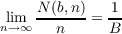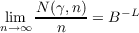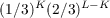#### Vol. 76, No. 1, 1978

 Recent Issues Vol. 323: 1  2 Vol. 322: 1  2 Vol. 321: 1  2 Vol. 320: 1  2 Vol. 319: 1  2 Vol. 318: 1  2 Vol. 317: 1  2 Vol. 316: 1  2Online Archive Volume: Issue:The Journal Subscriptions Editorial Board Officers Contacts Submission Guidelines Submission Form Policies for Authors ISSN: 1945-5844 (e-only) ISSN: 0030-8730 (print) Special Issues Author Index To Appear Other MSP Journals
Construction of generalized normal numbers

### F. J. Martinelli

Vol. 76 (1978), No. 1, 117–122
##### Abstract

Let x be a real number, 0 x < 1, and let 0.x1x2 be its expansion in the base B. Let N(b,n) be the number of occurrences of the digit b in x up to xn. Then x is called digit normal (in the base B) iffor each of the B possible values of b. Let γ be any fixed B-ary sequence of length L and N(γ,n) be the number of indices k for which xkxk+1xk+L1 is γ, that is, N(γ,n) is the number of times γ appears in the first n digits of x. Then x is normal (in the base B) iffor each of the BL possible sequences γ, and BL is called the limiting frequency of γ in x.

The purpose of this paper is to construct a generalized normal number (in the base 2) in which these frequencies are weighted. For example, we will obtain infinite binary decimals in which the limiting frequency of occurrence of ones is 1/3 (in general, p < 1) rather than 1/2; consequently, any binary string γ of length L will have limiting frequencywhere K is the number of ones in γ.

##### Mathematical Subject Classification
Primary: 10K25, 10K25
##### Milestones